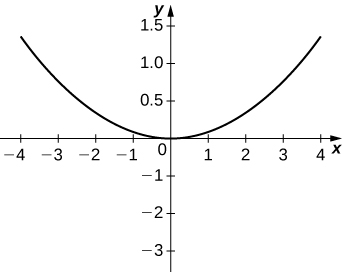$$\newcommand{\id}{\mathrm{id}}$$ $$\newcommand{\Span}{\mathrm{span}}$$ $$\newcommand{\kernel}{\mathrm{null}\,}$$ $$\newcommand{\range}{\mathrm{range}\,}$$ $$\newcommand{\RealPart}{\mathrm{Re}}$$ $$\newcommand{\ImaginaryPart}{\mathrm{Im}}$$ $$\newcommand{\Argument}{\mathrm{Arg}}$$ $$\newcommand{\norm}{\| #1 \|}$$ $$\newcommand{\inner}{\langle #1, #2 \rangle}$$ $$\newcommand{\Span}{\mathrm{span}}$$

11.5E: Exercises for Section 11.5

$$\newcommand{\vecs}{\overset { \rightharpoonup} {\mathbf{#1}} }$$ $$\newcommand{\vecd}{\overset{-\!-\!\rightharpoonup}{\vphantom{a}\smash {#1}}}$$$$\newcommand{\id}{\mathrm{id}}$$ $$\newcommand{\Span}{\mathrm{span}}$$ $$\newcommand{\kernel}{\mathrm{null}\,}$$ $$\newcommand{\range}{\mathrm{range}\,}$$ $$\newcommand{\RealPart}{\mathrm{Re}}$$ $$\newcommand{\ImaginaryPart}{\mathrm{Im}}$$ $$\newcommand{\Argument}{\mathrm{Arg}}$$ $$\newcommand{\norm}{\| #1 \|}$$ $$\newcommand{\inner}{\langle #1, #2 \rangle}$$ $$\newcommand{\Span}{\mathrm{span}}$$ $$\newcommand{\id}{\mathrm{id}}$$ $$\newcommand{\Span}{\mathrm{span}}$$ $$\newcommand{\kernel}{\mathrm{null}\,}$$ $$\newcommand{\range}{\mathrm{range}\,}$$ $$\newcommand{\RealPart}{\mathrm{Re}}$$ $$\newcommand{\ImaginaryPart}{\mathrm{Im}}$$ $$\newcommand{\Argument}{\mathrm{Arg}}$$ $$\newcommand{\norm}{\| #1 \|}$$ $$\newcommand{\inner}{\langle #1, #2 \rangle}$$ $$\newcommand{\Span}{\mathrm{span}}$$

In exercises 1 - 8, determine the equation of the parabola using the information given.

1) Focus $$(4,0)$$ and directrix $$x=−4$$

$$y^2=16x$$

2) Focus $$(0,−3)$$ and directrix $$y=3$$

3) Focus $$(0,0.5)$$ and directrix $$y=−0.5$$

$$x^2=2y$$

4) Focus $$(2,3)$$ and directrix $$x=−2$$

5) Focus $$(0,2)$$ and directrix $$y=4$$

$$x^2=−4(y−3)$$

6) Focus $$(−1,4)$$ and directrix $$x=5$$

7) Focus $$(−3,5)$$ and directrix $$y=1$$

$$(x+3)^2=8(y−3)$$

8) Focus $$\left(\frac{5}{2},−4\right)$$ and directrix $$x=\frac{7}{2}$$

In exercises 9 - 16, determine the equation of the ellipse using the information given.

9) Endpoints of major axis at $$(4,0),\;(−4,0)$$ and foci located at $$(2,0),\;(−2,0)$$

$$\dfrac{x^2}{16}+\dfrac{y^2}{12}=1$$

10) Endpoints of major axis at $$(0,5),\;(0,−5)$$ and foci located at $$(0,3),\;(0,−3)$$

11) Endpoints of major axis at $$(0,2),\;(0,−2)$$ and foci located at $$(3,0),\;(−3,0)$$

$$\dfrac{x^2}{13}+\dfrac{y^2}{4}=1$$

12) Endpoints of major axis at $$(−3,3),\;(7,3)$$ and foci located at $$(−2,3),\;(6,3)$$

13) Endpoints of major axis at $$(−3,5),\;(−3,−3)$$ and foci located at $$(−3,3),\;(−3,−1)$$

$$\dfrac{(y−1)^2}{16}+\dfrac{(x+3)^2}{12}=1$$

14) Endpoints of major axis at $$(0,0),\;(0,4)$$ and foci located at $$(5,2),\;(−5,2)$$

15) Foci located at $$(2,0),\;(−2,0)$$ and eccentricity of $$\frac{1}{2}$$

$$\dfrac{x^2}{16}+\dfrac{y^2}{12}=1$$

16) Foci located at $$(0,−3),\;(0,3)$$ and eccentricity of $$\frac{3}{4}$$

In exercises 17 - 24, determine the equation of the hyperbola using the information given.

17) Vertices located at $$(5,0),\;(−5,0)$$ and foci located at $$(6,0),\;(−6,0)$$

$$\frac{x^2}{25}−\frac{y^2}{11}=1$$

18) Vertices located at $$(0,2),\;(0,−2)$$ and foci located at $$(0,3),\;(0,−3)$$

19) Endpoints of the conjugate axis located at $$(0,3),\;(0,−3)$$ and foci located $$(4,0),\;(−4,0)$$

$$\dfrac{x^2}{7}−\dfrac{y^2}{9}=1$$

20) Vertices located at $$(0,1),\;(6,1)$$ and focus located at $$(8,1)$$

21) Vertices located at $$(−2,0),\;(−2,−4)$$ and focus located at $$(−2,−8)$$

$$\dfrac{(y+2)^2}{4}−\dfrac{(x+2)^2}{32}=1$$

22) Endpoints of the conjugate axis located at $$(3,2),\;(3,4)$$ and focus located at $$(3,7)$$

23) Foci located at $$(6,−0),\;(6,0)$$ and eccentricity of $$3$$

$$\dfrac{x^2}{4}−\dfrac{y^2}{32}=1$$

24) $$(0,10),\;(0,−10)$$ and eccentricity of 2.5

In exercises 25 - 30, consider the following polar equations of conics. Determine the eccentricity and identify the conic.

25) $$r=\dfrac{−1}{1+\cos θ}$$

$$e=1,$$ parabola

26) $$r=\dfrac{8}{2−\sin θ}$$

27) $$r=\dfrac{5}{2+\sin θ}$$

$$e=\frac{1}{2},$$ ellipse

28) $$r=\dfrac{5}{−1+2\sin θ}$$

29) $$r=\dfrac{3}{2−6\sin θ}$$

$$e=3$$, hyperbola

30) $$r=\dfrac{3}{−4+3\sin θ}$$

In exercises 31 - 34, find a polar equation of the conic with focus at the origin and eccentricity and directrix as given.

31) Directrix: $$x=4;\; e=\frac{1}{5}$$

$$r=\dfrac{4}{5+\cos θ}$$

32) Directrix: $$x=−4;\; e=5$$

33) Directrix: $$y=2; \; e=2$$

$$r=\dfrac{4}{1+2\sin θ}$$

34) Directrix: $$y=−2;\; e=\frac{1}{2}$$

In exercises 35 - 51, sketch the graph of each conic.

35) $$r=\dfrac{1}{1+\sin θ}$$36) $$r=\dfrac{1}{1−\cos θ}$$

37) $$r=\dfrac{4}{1+\cos θ}$$38) $$r=\dfrac{10}{5+4\sin θ}$$

39) $$r=\dfrac{15}{3−2\cos θ}$$40) $$r=\dfrac{32}{3+5\sin θ}$$

41) $$r(2+\sin θ)=4$$42) $$r=\dfrac{3}{2+6\sin θ}$$

43) $$r=\dfrac{3}{−4+2\sin θ}$$44) $$\dfrac{x^2}{9}+\dfrac{y^2}{4}=1$$

45) $$\dfrac{x^2}{4}+\dfrac{y^2}{16}=1$$46) $$4x^2+9y^2=36$$

47) $$25x^2−4y^2=100$$48) $$\dfrac{x^2}{16}−\dfrac{y^2}{9}=1$$

49) $$x^2=12y$$50) $$y^2=20x$$

51) $$12x=5y^2$$For the equations in exercises 52 - 57, determine which of the conic sections is described.

52) $$xy=4$$

53) $$x^2+4xy−2y^2−6=0$$

Hyperbola

54) $$x^2+2\sqrt{3}xy+3y^2−6=0$$

55) $$x^2−xy+y^2−2=0$$

Ellipse

56) $$34x^2−24xy+41y^2−25=0$$

57) $$52x^2−72xy+73y^2+40x+30y−75=0$$

Ellipse

58) The mirror in an automobile headlight has a parabolic cross section, with the lightbulb at the focus. On a schematic, the equation of the parabola is given as $$x^2=4y$$. At what coordinates should you place the lightbulb?

59) A satellite dish is shaped like a paraboloid of revolution. The receiver is to be located at the focus. If the dish is 12 feet across at its opening and 4 feet deep at its center, where should the receiver be placed?

At the point 2.25 feet above the vertex.

60) Consider the satellite dish of the preceding problem. If the dish is 8 feet across at the opening and 2 feet deep, where should we place the receiver?

61) A searchlight is shaped like a paraboloid of revolution. A light source is located 1 foot from the base along the axis of symmetry. If the opening of the searchlight is 3 feet across, find the depth.

$$0.5625$$ feet

62) Whispering galleries are rooms designed with elliptical ceilings. A person standing at one focus can whisper and be heard by a person standing at the other focus because all the sound waves that reach the ceiling are reflected to the other person. If a whispering gallery has a length of 120 feet and the foci are located 30 feet from the center, find the height of the ceiling at the center.

63) A person is standing 8 feet from the nearest wall in a whispering gallery. If that person is at one focus and the other focus is 80 feet away, what is the length and the height at the center of the gallery?

Length is 96 feet and height is approximately 26.53 feet.

In exercises 64 - 67, determine the polar equation form of the orbit given the length of the major axis and eccentricity for the orbits of the comets or planets. Distance is given in astronomical units (AU).

64) Halley’s Comet: length of major axis = $$35.88,$$ eccentricity = $$0.967$$

65) Hale-Bopp Comet: length of major axis = $$525.91,$$ eccentricity = $$0.995$$

$$r=\dfrac{2.616}{1+0.995\cos θ}$$

66) Mars: length of major axis = $$3.049,$$ eccentricity = $$0.0934$$

67) Jupiter: length of major axis = $$10.408,$$ eccentricity = $$0.0484$$

$$r=\dfrac{5.192}{1+0.0484\cos θ}$$